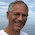## tisdag 3 februari 2015

### Physical Quantum Mechanics 8: Two-Electron Ions: Theory vs Observation

The atomic model studied in this sequence of posts (based on the sequence on Quantum Contradictions) takes the following form for the ground state of a two-electron ion: Find two real-valued wave functions $\psi_1(x)$ and $\psi_2(x)$ depending on a 3d space coordinate $x=(x_1,x_2,x_3)$ with non-overlapping supports, such that the function $\psi (x)=\psi_1(x)+\psi_2(x)$ minimizes the energy functional with integration over 3d space
• $\frac{1}{2}\int\vert\nabla\psi\vert^2 dx-\int\frac{N\psi^2}{\vert x\vert}dx+\int\int\frac{\psi_1^2(x)\psi_2^2(y)}{\vert x-y\vert}dxdy$,
under the condition of charge conservation:
• $\int\psi_j^2(x)dx =1$ for $j=1,2$.
We are led to an approximate minimizer formed by the following half-spherically symmetric wave functions supported by two half-spaces filling 3d space
• $\psi_1(x)=\sqrt{\frac{2\alpha^3}{\pi}}\exp(-\alpha\vert x\vert )$ for $x=(x_1,x_2,x_3)$ with $x_3\ge 0$
• $\psi_2(x)=\sqrt{\frac{2\alpha^3}{\pi}}\exp(-\alpha\vert x\vert )$ for $x$ with $x_3\le 0$,
where $\alpha =N - 5/16$. Computing the first two integrals of the energy functional analytically and the third approximately by quadrature, we obtain the following expression for the ground state energy:
• $E(N)= \alpha^2 -2N\alpha + \frac{\alpha}{2-\frac{5}{16}}1.00$.
We obtain the following values with experimentally observed values in parenthesis:
• $E(2) = -2.903$ for Helium ($-2.903$)
• $E(3)= -7.31$ for Li+ ($-7.28$)
• $E(4)= -13.72$ for Be2+ ( $-13.65$)
• $E(5) = -22.12$ for B3+ ( $-22.03$)
• $E(6)= -32.53$ for C4+ ($-32.41$)
• $E(7)= -44.94$ for N5+ ($-44.80$)
• $E(8)=-59.35$ for O6+ ($-59.19$)
• $E(9)=-75.75$ for F7+ ($-75.60$)
We see a surprisingly good agreement within 0.1%. It is possible that with more precise quadrature the agreement is even better.

We may compare with the postulated standard 1s2 ground state of Helium consisting of two overlaying fully spherical wave functions, which gives a best value of -2.85 asking for so called perturbation correction, which effectively introduces electron separation.

We have thus found evidence that the ground state of Helium is not the standard 1s2 state with two overlaying spherical wave functions, without or with perturbation correction, but instead consists of two non-overlapping half-spherical wave functions. For illuminating graphics see here.

We thus consider a 3d 2-electron wave function $\psi (x) =\psi_1(x)+\psi_2(x)$ as a sum of two 3d 1-electron wave functions $\psi_1(x)$ and $\psi_2(x)$ with non-overlapping support, with clear physical meaning.

Such a wave function can be approximated in the standard multi-variable form $\psi (x,y)$ depending on a 6d space coordinate $(x,y)$, however without direct physical meaning. It is thus no wonder that a perturbation computation starting from a 1s2 configuration can have ground state energy in correspondence with observation, while the 1s2 configuration has not.

But if already the physical 3d 2-electron wave function gives good correspondence with observation, as we have seen, then there is no rational reason to seek approximations as 6d unphysical wave functions.  If this turns out to be correct, then quantum mechanics will require revision.

PS The ground-state can be computed by parabolic relaxation by time-stepping the system
• $\dot\psi_1 + H_1\psi_1=0$
• $\dot\psi_2 + H_2\psi_2=0$,
where
• $H_1 =-\frac{1}{2}\Delta -\frac{N}{\vert x\vert}+\int_{y_3 < 0}\frac{\psi_2^2(y)}{\vert x-y\vert}dy$ for $x_3> 0$
• $H_2 =-\frac{1}{2}\Delta -\frac{N}{\vert x\vert}+\int_{y_3 > 0}\frac{\psi_1^2(y)}{\vert x-y\vert}dy$ for $x_3< 0$
with rescaling in each time-step to conserve charge, and with the free-boundary fixed at $x_3=0$, starting e.g. from the above wave functions.

#### 2 kommentarer:

1.Thank you for a very interesting and understandable series of posts on Physical Quantum Mechanics.
After having struggled with David Bohm's "Quantum Theory" for a while and never found a reasonable explanation for the initial choice of a complex first order mathematical model for the wave function, it is a relief for an engineer working practically with structural and fluid dynamics, to read your analysis of the alternative model, also proposed by Schroedinger, leading to well-known second order real-valued models.

2.Thank you for your interest!